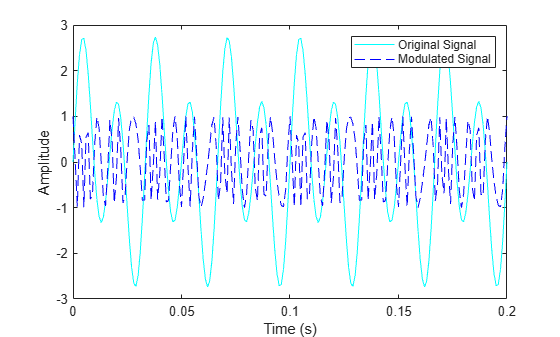# fmmod

Frequency modulation

## Syntax

``y = fmmod(x,Fc,Fs,freqdev)``
``y = fmmod(x,Fc,Fs,freqdev,ini_phase)``

## Description

example

````y = fmmod(x,Fc,Fs,freqdev)` returns a frequency modulated (FM) signal `y`, given the input message signal `x`, where the carrier signal has frequency `Fc` and sampling rate `Fs`. `freqdev` is the frequency deviation of the modulated signal. Note The value of `Fs` must satisfy `Fs` ≥ 2`Fc`.The value of `freqdev` must satisfy `freqdev` < `Fc`. ```
````y = fmmod(x,Fc,Fs,freqdev,ini_phase)` specifies the initial phase of the modulated signal.```

## Examples

collapse all

Set the sampling frequency to 1kHz and carrier frequency to 200 Hz. Generate a time vector having a duration of 0.2 s.

```fs = 1000; fc = 200; t = (0:1/fs:0.2)';```

Create two tone sinusoidal signal with frequencies 30 and 60 Hz.

`x = sin(2*pi*30*t)+2*sin(2*pi*60*t);`

Set the frequency deviation to 50 Hz.

`fDev = 50;`

Frequency modulate `x`.

`y = fmmod(x,fc,fs,fDev);`

Plot the original and modulated signals.

```plot(t,x,'c',t,y,'b--') xlabel('Time (s)') ylabel('Amplitude') legend('Original Signal','Modulated Signal')```## Input Arguments

collapse all

Input message signal, specified as a scalar, vector, matrix, or a 3-D array. Each element of `x` must be real.

Data Types: `single` | `double`

Carrier frequency in hertz (Hz), specified as a positive real scalar.

Data Types: `single` | `double`

Sampling rate in hertz (Hz), specified as a positive real scalar.

Data Types: `single` | `double`

Frequency deviation of the modulated signal in hertz (Hz), specified as a positive real scalar.

Data Types: `single` | `double`

Initial phase of the modulated signal in radians, specified as a real scalar.

Data Types: `single` | `double`

## Output Arguments

collapse all

Frequency modulated signal, returned as a scalar, vector, matrix, or 3-D array.

## Version History

Introduced before R2006a